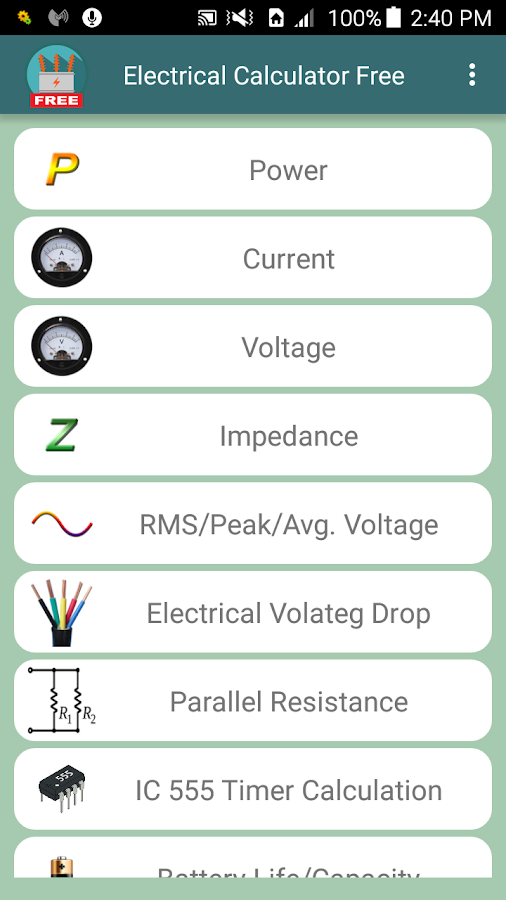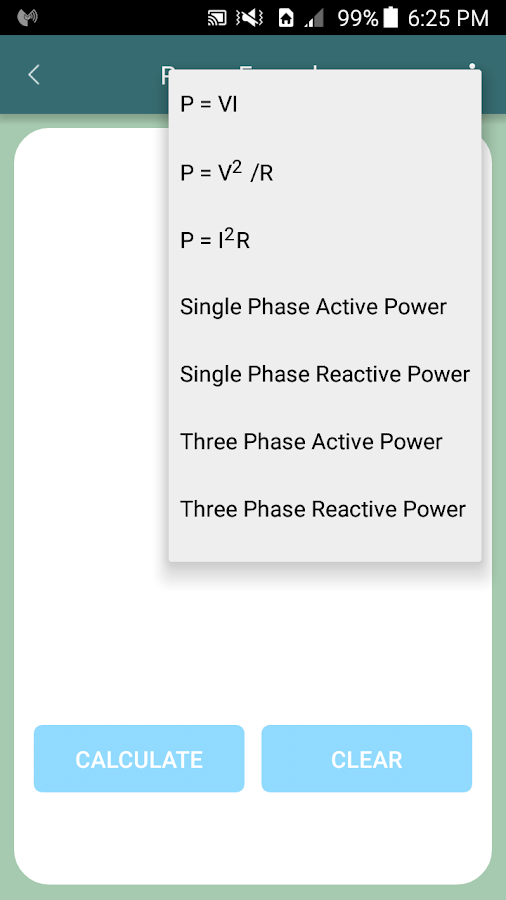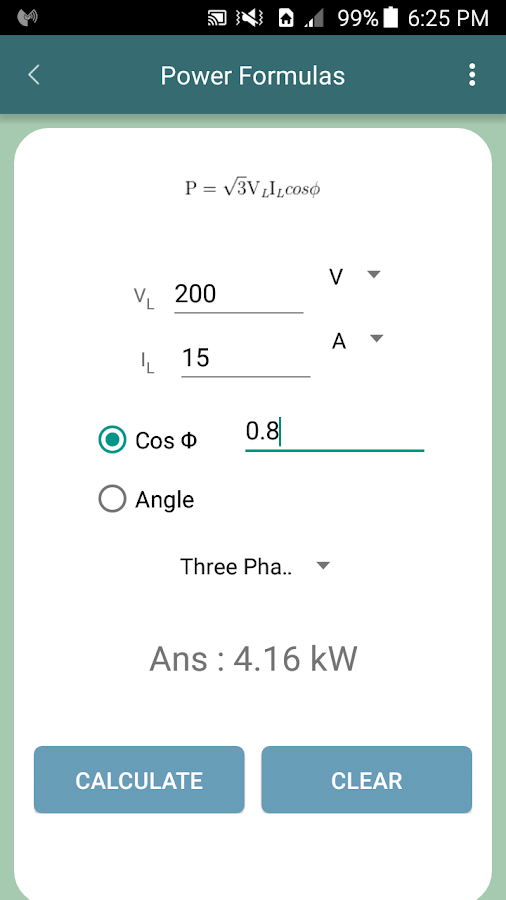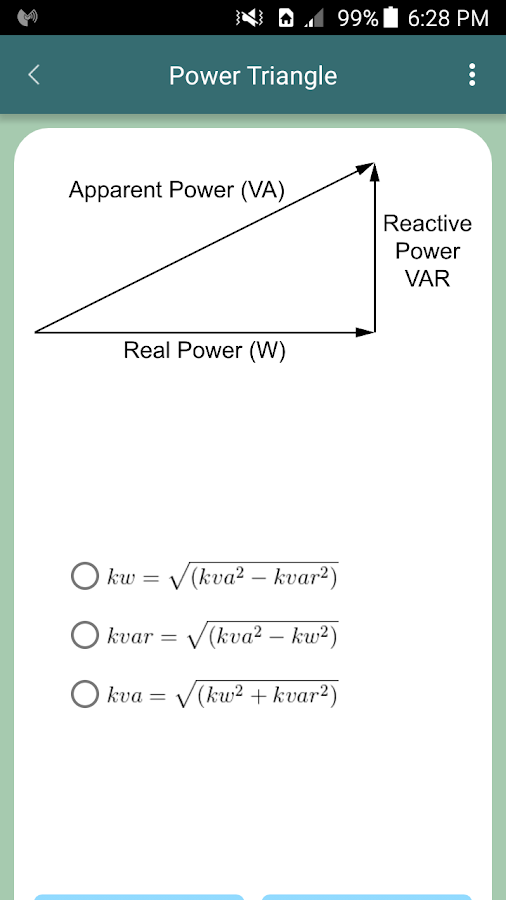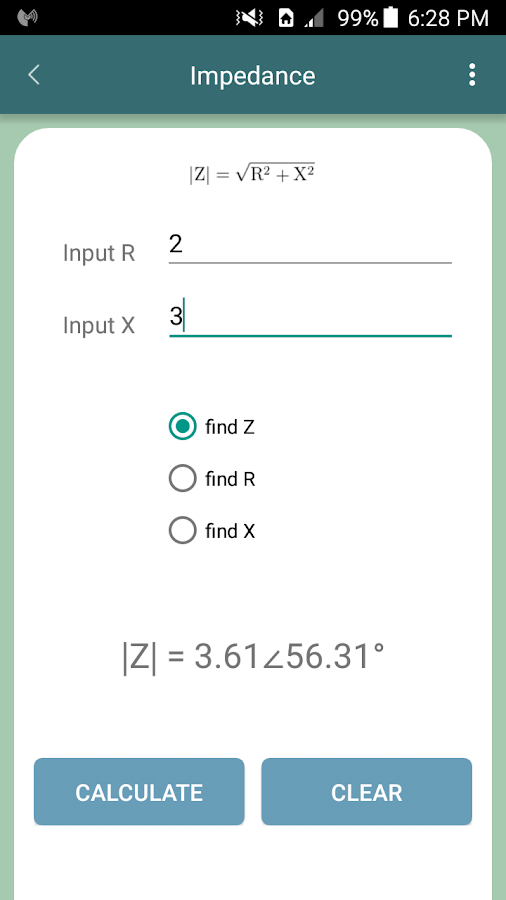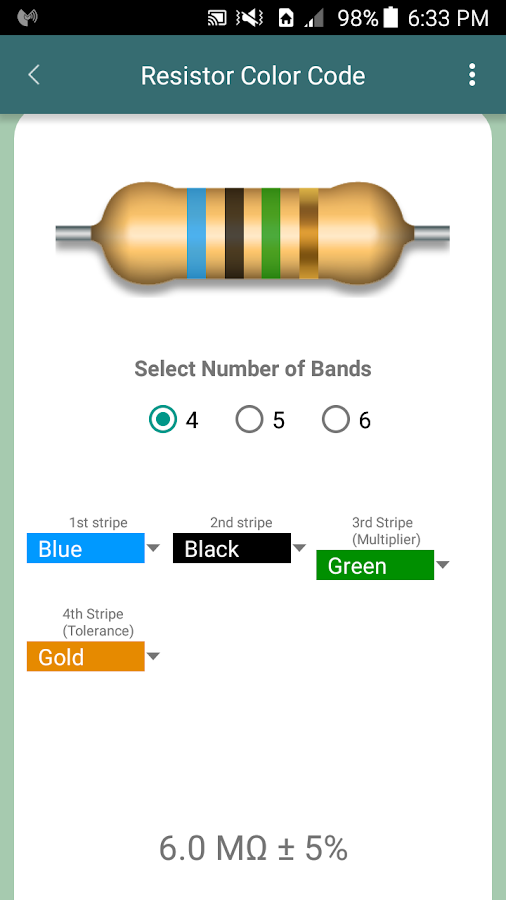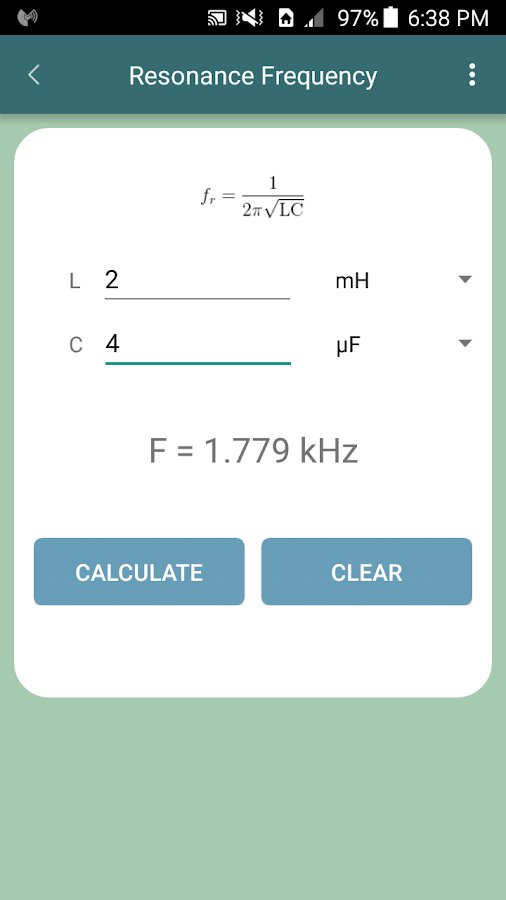General Electrical Calculator Free

Discussion in 'Android Apps & Games' started by Bikram Saha Ray, Apr 11, 2016.

1. Bikram Saha Ray Lurker Thread Starter Rank: None Points: 5 Posts: 1 Joined: Apr 11, 2016

Apr 11, 2016
1
0
5
Male
This app is created for Electrical Engineers and Engineering students who encounter various numerical problems in their day to day activity. This app helps to solve complex mathematical problems in an easy manner. It helps to save both time and effort while providing accurate results to various calculations.

This app features various common equations as well as several difficult and complex equations such as those of Transformers, Induction Motor, Power system e.t.c, encountered by electrical engineering students during their course. Almost all sections show the relevant equations involved and attempts to solve them. There is also a "Labels" section that describe the parameters and variables used in the equation. In addition to that a Help section is added to aid users.

Here is a list of calculators provided in the App :

* Power

• Power formulas
• Power Triangle

* Current

• Current formulas
• Current Rectangular to Polar co-ordinates converter

* Voltage

• Voltage formulas
• Voltage Rectangular to Polar co-ordinates converter
• Voltage Divider

* Impedance

* RMS/Peak/ Average Converter

* Electrical Voltage Drop

* Parallel Resistance

* IC 555 Timer Calculation

* Battery Life/Capacity Calculator

* Star-Delta Converter

* Electrical Reactance

* Resonance Frequency

* Transformer (Pro Version Only)

* DC Motor/Generator (Pro Version Only)

* Induction Motor (Pro Version Only)

* Alternator (Pro Version Only)

* Power system and Switch gear (Pro Version Only)

* Electrical Unit Converter

* 8085 Pinout Diagram

* Resistor Color Code

* Inductor Color Code

* SMD Resistor Code

* Power Factor Correction

More sections will be added from time to time